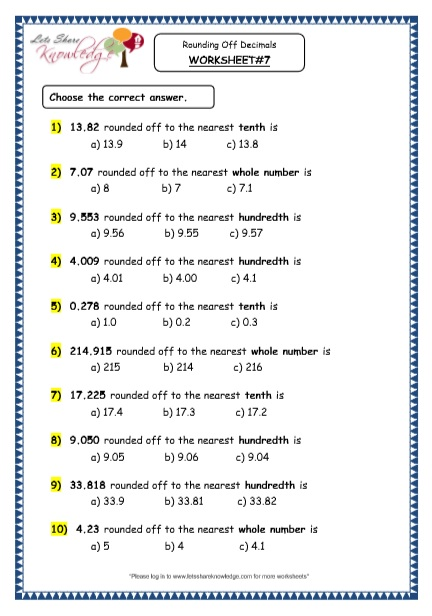Jan 9, 2018
7985 Views

# Grade 4 Maths Resources (3.4 Rounding Off Decimals Printable Worksheets)# Rounding Off Decimals Printable Worksheets

Rounding Off Decimals Printable Worksheets – Rules to round off a decimal number are same like rounding off whole numbers.

Let’s take an example to review the rules.

Example: 3 5 . 3 8 7

Round off to the nearest whole number:

To round off to the nearest whole number, we’ll look at the digit at the tenths place. If its <5 we’ll round it down and if its >5 we’ll round it up.

Here, since 3 is at tenths place and 3<5 so the answer will be 35!

Round off to the nearest tenth:

To round off to the nearest tenth, we’ll look at the digit at the hundredths place. If its <5 we’ll round it down and if its >5 we’ll round it up.

Here, since 9 is at hundredths place and 9>5 so the answer will be 35.4!

Round off to the nearest hundredth:

To round off to the nearest hundredth, we’ll look at the digit at the thousandths place. If its <5 we’ll round it down and if its >5 we’ll round it up.

Here, since 7 is at thousandth place and 7>5 so the answer will be 35.39!

.